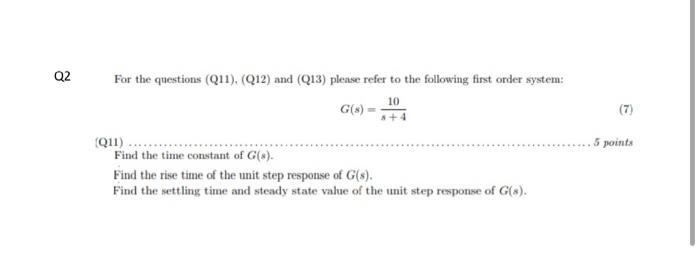# Question For the questions (Q11), (Q12) and (Q13) please refer to the following first order system: $G(s)=\frac{10}{s+4}$ (Q)1) Find the time constant of $$G(s)$$. Find the rise time of the unit step response of $$G(s)$$. Find the settling time and steady state value of the unit step response of $$G(s)$$.VO54QF The Asker · Mechanical EngineeringTranscribed Image Text: For the questions (Q11), (Q12) and (Q13) please refer to the following first order system: $G(s)=\frac{10}{s+4}$ (Q)1) Find the time constant of $$G(s)$$. Find the rise time of the unit step response of $$G(s)$$. Find the settling time and steady state value of the unit step response of $$G(s)$$.
More
Transcribed Image Text: For the questions (Q11), (Q12) and (Q13) please refer to the following first order system: $G(s)=\frac{10}{s+4}$ (Q)1) Find the time constant of $$G(s)$$. Find the rise time of the unit step response of $$G(s)$$. Find the settling time and steady state value of the unit step response of $$G(s)$$.&#12304;General guidance&#12305;The answer provided below has been developed in a clear step by step manner.Step1/2The first order transfer function is given:$$\mathrm{{G}{\left({s}\right)}=\frac{{10}}{{{s}+{4}}}{\quad\text{or}\quad}\frac{{2.5}}{{{0.25}{s}+{1}}}}$$Above transfer function compare with, $$\mathrm{\frac{{K}}{{\tau{s}+{1}}}}$$Here, $$\mathrm{\tau}$$is a time constant$$\mathrm{\tau={0.25}{\sec{}}}$$$$\mathrm{{K}}$$is a DC gain or steady state gain$$\mathrm{{K}={2.5}}$$Explanation:Please refer to solution in this step.Step2/2Define the rise time of the unit step response of $$\mathrm{{G}{\left({s}\right)}}$$Where, \( ... See the full answer